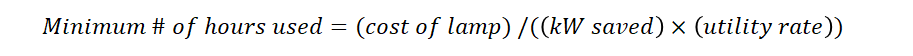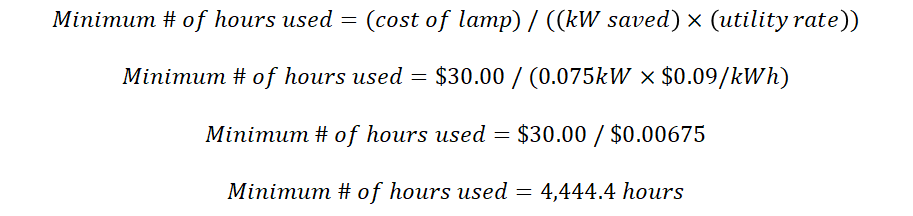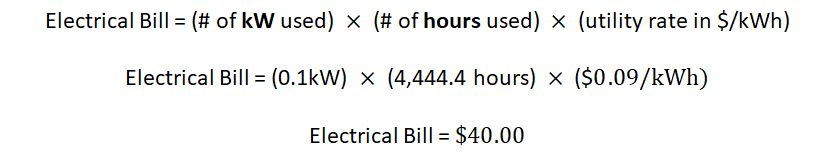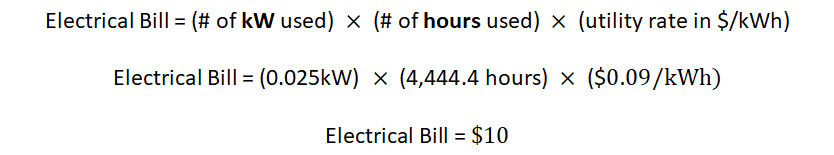# 24 Energy Cost Example 3 – How long to pay for itself?

Aaron Lee

How many hours does the LED need to be in operation before it pays for itself in energy savings?

People are naturally hesitant to change from familiar lighting systems, especially if the initial cost of the new lighting system is more expensive. One of the ways to put these costs in perspective is to calculate how many hours the new system needs to be in use before it pays for itself in reduced energy bills.

The minimum number of hours in use can be calculated with this formula:A 25-watt energy-efficient LED lamp is to replace a 100 W incandescent lamp of the same lumen output. If the cost of the LED is \$30.00 to purchase, and the utility rate is \$0.09 per kWh used, after how many hours will the LED have paid for itself?

Since the LED is rated at 25 watts compared to the 100 watts used by the incandescent lamp, this represents an energy savings of 75 watts. By multiplying these saved watts by the utility rate, we can calculate how much money we are saving for every hour in use.After roughly 4,444 and a half hours, or just over 185 days, which is roughly 6 months, the LED lamp has paid for itself with a reduced electric bill.

Recalculate the costs of running each of the two lighting systems for the calculated minimum number of hours to confirm our results:

Incandescent electrical costs:LED electrical costs:After six months of use, the LED lamp has saved \$30.00 in electrical utility costs and has thus repaid its own initial investment cost of \$30.00.

This type of calculation can be used for any energy-efficient equipment that is proposed to replace an older system.